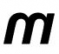## FDAToolStarted by 7 years ago9 replieslatest reply 7 years ago592 views

I am new to FDATool (#matlab) and would like to use it to generate an impulse response with complex coefficients.

I would like to low pass filter the signal after IFFT in #OFDM and the signal is complex. So am unsure how to filter a complex signal?

Thanks,

[ - ]I have never used FDATool, it looks like it expects a single real signal for input.  If your output is complex, I assume that means it is a quadrature signal.

Your output is I and Q.  Just treat them as separate "real" signals and sent each one through the same filter you design with the FDATool.  Use the tool to design a filter for one real input - and then use it twice.

Patience, persistence, truth,

Dr. mike

[ - ]Thanks Dr. Let me try.

[ - ]To echo Dr. mike's good advice: if your FDAtool produces filter coefficients, h, whose performance is what you desire, then you should use the h coefficients filter the real part of your complex-valued input signal to produce the real part of your filter output signal. Likewise, using the same filter coefficients, filter the imaginary part of your complex-valued input signal to produce the imaginary part of your filter output signal.

But LabPE43, MATLAB's 'filter(h, 1, Input_Signal)' command will perform this dual-filtering operation automatically! The 'filter(h, 1, Input_Signal)' command examines it's "Input_Signal" input sequence and if "Input_Signal" is complex-valued the 'filter()' command filters the input's real and imaginary parts separately and produces a complex-valued output. What I'm saying is, MATLAB will perform your complex filtering with just a single command such as:

Output_Signal = filter(h, 1, Input_Signal)

and the desired "Output_Signal" sequence will be complex-valued.

[ - ]Thanks for the valuable information. Sometimes, its cost effective to perform filtering in frequency instead of time domain.

When input signal is larger than the length of the impulse response, the impulse response is zero padded for equal length. So how to form the complex version of the impulse response?  Do I need to combine the real impulse response to form complex and then take its FFT like

FFT(h+jh) = Hfreq.

Output = FFT(Input_Signal) x Hfreq

I could try in MatLab but just wanna confirm theory is correct.

I may not have the option to use filter().

[ - ]LabPe43, to answer your last question, if freq-domain filtering is what you desire then the first step is to merely compute the FFT of the 'h' coefficients as:

Hfreq = FFT(h);

Hfreq will be complex-valued. Next, compute spectrum of your Input_Signal as:

Spec_Input = FFT(Input_Signal);

The time-domain filtered sequence is the inverse FFT of the freq-domain product as:

Output = Inverse_FFT[Hfreq x Spec_Input);

[ - ]Dr Mike is giving good advice. The resolved I,Q components which form a complex vector signal are orthogonal. This property allows them to be filtered by IDENTICAL scalar filters. You can design the filters by any convenient method.

[ - ]I'm trying to think of why one would want to do what you're suggesting -- either my understanding of OFDM is insufficient (and it _is_ sketchy), or you're doing something that is neither necessary or helpful.

Could you go over your rationale for this low-pass filtering?  It seems like all you'd be doing would be losing data.

[ - ]Dear Tim Wescott,

Its filtered OFDM I would like to work. Sub-band filtering is required to filter out UE's sub-band. Hope this explains.

Thank you all for clearing my confusion about I and Q filtering.

[ - ]